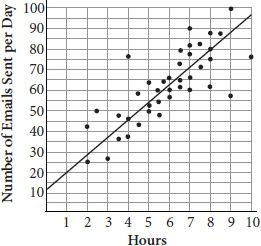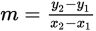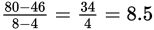# SAT Math Multiple Choice Question 850: Answer and Explanation

### Test Information

Question: 850

10.The figure above shows the number of emails sent per day plotted against the numberof hours an adult works on a computer per day. Which of the following best estimatesthe average rate of change in the number of emails sent compared to the number ofhours working on a computer?

• A. 0.12
• B. 1.5
• C. 8.5
• D. 12

Getting to the Answer: Find the slope of the best-fit line using the slope formula,, and any two points that lie on (or very close to) the line. Using the two points (4, 46) and (8, 80), the average rate of change is about, which is (C). Note that the answer choices are spread out enough that any two points along the line, even points for which you estimate the coordinates, will yield a slope that is close enough to one of the answer choices.